# Neural Network Architectures

## From Probabilistic Deep Learning with Python by Oliver Dürr, Beate Sick, and Elvis Murina

This article dives into neural network architectures and how get started implementing and using them.

___________________________________________________________

Take 37% off Probabilistic Deep Learning with Python by entering fccdurr into the discount code box at checkout at manning.com.

___________________________________________________________

Before diving into the details of the different DL architectures, let’s look at figure 1 and consider the architecture of a typical traditional artificial NN. The visualized NN has three hidden layers, each holding nine neurons. Each neuron within a layer is connected with each neuron in the next layer. This is the reason why this architecture is called a densely connected NN or a fully connected neural network (fcNN).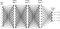Figure 1 An example of a fully connected neural network (fcNN) model with three hidden layers

The design of NNs is inspired by the way the brain works. You shouldn’t overstretch this point; it’s a loose inspiration. The brain is a network of neurons. The human brain has about one hundred billion neurons, and each neuron is, on average, connected with ten thousand other neurons. Let’s take a look at the brain’s basic unit — a neuron.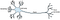Figure 2 A single biological brain cell. The neuron receives the signal from other neurons via its dendrites shown on the left. If the cumulated signal exceeds a certain value, an impulse is sent via the axon to the axon terminals, which, in turn, couples to other neurons.

Figure 2 shows a simplified sketch of a neuron. It receives signals from other neurons via its dendrites. Some inputs have an activating impact, and some inputs have an inhibiting impact. The received signal is accumulated and processed within the cell body of the neuron. If the signal is strong enough, the neuron fires. That means it produces a signal which is transported to the axon terminals. Each axon terminal connects to another neuron. Some connections can be stronger than others, which makes it easier to transduce the signal to the next neuron. The strength of these connections can be changed by experiences and learning. Computer scientists have derived a mathematical abstraction from the biological brain cell, the artificial neuron shown in figure 3.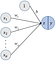Figure 3 The mathematical abstraction of a brain cell (an artificial neuron). The value z is computed as the weighted sum of the p input values, x1 to xp, and a bias term b that shifts up or down the resulting weighted sum of the inputs. The value y is computed from z by applying an activation function.

An artificial neuron receives some numeric input values, x1, which are multiplied with some corresponding numeric weights, w1 , The inputs are accumulated by determining the weighted sum of the inputs plus a bias term, b (which gets 1 as input), as z=x1 ∙ w1+x1 ∙ w1++ xp ⋅ wp+1 ⋅ b..Note that this formula is the same as that used in linear regression. The resulting z is then further transformed by a non-linear activation function, the so-called sigmoid function, which transfers the number z to a number between 0 and 1 (see figure 2.4). This function is given by:

As you can see in figure 4, large positive values of z result in values close to one, and negative values with large absolute values result in values close to zero. In this sense, the resulting value y can be interpreted as the probability that the neuron fires. Or, in the context of classification, as a probability for a certain class. If you want to build a binary classifier (with 0 and 1 as possible classes) that takes several numeric features xi and generates the probability for class one, then you can use a single neuron. If you’ve a background in statistics, this might look familiar and, indeed, a network with a single neuron’s known in statistics also as logistic regression. No worries if you never heard of logistic regression.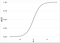Figure 4 The sigmoid function f translating (squeezing) an arbitrary number z to a number between 0 and 1.

To get you started working with deep learning, you need to know the basic data structures, the tensors and the software packages manipulating those entities.

Looking at figure 3, the mathematical abstraction of a neuron, you might ask the question “What goes in and what comes out”? Assuming that p = 3 in figure 3, then you see three numbers,x1, x2 and x3 entering the neuron and a single number leaving the neuron. These three numbers can be treated as an array with one index. More complex neural networks can take a grayscale image, say of size 64 x 32 as input, which can be also expressed as an array. But this time the array has two indexes. The first index, i, ranges from 0 to 63 and the second, j, from 0 to 31.

Going further, say you have a color image with the colors red, green, and blue. For such an image, each pixel has x,y coordinates and three additional values. The image can be stored in an array with three indices (i,j,c). Taking it to the more extreme, say you input a whole stack of 128 color images into the network. These could be stored in an array of (b,x,y,c) with b ranging from 0 to 127. Also, the three weights in figure 3 can be viewed as an array with one index, going from 0 to 2.

As it turns out, all quantities in DL can be put into arrays. In the context of DL, these arrays are called tensors, and from an abstract standpoint, all that happens in DL is the manipulation of tensors. The number of indices tensors have is the dimension, order, or sometimes rank (don’t get confused). Tensors of order zero, like the output of the neuron in figure 3, have no indices. Tensors with low orders also have special names:

• Tensors of order zero are called scalars.
• Tensors of order one are called vectors.
• Tensors of order two are called matrices.

The shape of a tensor defines how many values each index can have. For example, if you have a gray-valued image of 64 x 32 pixels, the shape of the tensor would be (64,32). This is all you need to know about tensors when you use DL. Be aware when you google tensors, you might find frightening stuff, like the mathematical definition by its transformation properties. Don’t worry, in the context of DL, a tensor is only a data container with a special structure like, for example, a vector or a matrix.

DL has gained enormous popularity with the availability of software frameworks which are built to manipulate tensors. In this article, we mainly use Keras (https://keras.io/) and TensorFlow (https://www.tensorflow.org/). These are the two frameworks currently most often used by DL partitioners. TensorFlow is an open source framework developed by Google that comes with strong support for DL. Keras is a user friendly, high-level neural networks API, written in Python, and capable of running on top of TensorFlow, allowing for fast prototyping.

To work through the exercises in this article, we recommend that you use the Google Colab environment (https://colab.research.google.com) as a cloud solution that runs in your browser. The most important frameworks, packages, and tools for DL are already installed, and you can immediately start coding. If you want to install a DL framework on your own computer, we recommend you follow the description given in https://livebook.manning.com/#!/book/deep-learning-with-python/chapter-3/73

We use Jupyter notebooks (https://jupyter.org/) to provide you with some hands-on exercises and code examples. Jupyter notebooks offer the ability to mix Python, TensorFlow, and Keras code with text and markdown. The notebooks are organized in cells containing either text or code. This lets you play around with the code by changing only the code in one cell. In many exercises, we provide large parts of the code, and you can experiment in individual cells with your own code. Feel free to also change the code at any location; you can’t break anything. Although deep learning often involves huge data and needs enormous compute power, we distilled simple examples to allow you to interactively work with the notebooks.

We use the following icon to indicate the positions in the article where you should open a Jupyter notebook and work through the related code:

You can open these notebooks directly in Google Colab, where you can edit and run these in your browser. Colab is great, though you need to be online to use it. Another option (good for working offline) is to use the provided Docker container; see https://tensorchiefs.github.io/dl_book/ for details on how to install Docker.

Within the Jupyter notebooks, we used the following icon to indicate where you should return to the book:

Let’s make it concrete and do a first experiment. In this experiment, you use a single artificial neuron to discriminate real from fake banknotes.

Open https://github.com/tensorchiefs/dl_book/blob/master/chapter_02/nb_ch02_01.ipynb, where you’ll find a data set describing 1,372 banknotes by two features and a class label y.

The two image features are based on wavelet analysis, a frequently used method in traditional image analysis. It’s common to store the input values and the target values in two separate tensors. The input data set contains 1,372 instances described by two features that you can organize in one 2D tensor. The first dimension usually describes the samples. This axis is referred to as axis 0. For the example, you’ve a 2D tensor with shape (1372,2). The target values are the true class labels that can be stored in a second 1D tensor with shape (1372).

DL models typically run on graphic cards also called graphic processing units (GPUs). These GPUs have limited memory; therefore you can’t process an entire data set at once. The data is split into smaller batches containing only a subset of the entire data set. These batches are called mini-batches, and a typical number of instances contained in a mini-batch is either 32, 64, or 128. In our banknote example, we use mini-batches with a size shape 128.

Because the banknotes are described by only two features, you can easily see the positions of real and fake banknotes in the 2D feature space shown in figure 5 and that the boundary between the two classes isn’t separable by a straight line.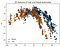Figure 5 The (training) data points for the real and fake banknotes

Let’s use a single neuron with a sigmoid activation function (also known as logistic regression) as a classification model (see figure 6) to separate fake from real banknotes for the data shown in figure 5.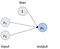Figure 6 An fcNN with one single neuron. The two nodes in the input layer correspond to the two features describing each banknote. The output layer has one node that corresponds to the probability of class one (fake banknote).

Before we define the Keras code, let’s think of the tensor structure needed. What goes into the network? If you use a single training data point, it’s a vector with two entries (the next section discusses how the bias is handled). If you take a batch of size 128 of those vectors, you’ve a tensor of order two (a matrix) with the shape (128,2). Usually one doesn’t specify the batch size when defining the network. In that case, you use None as the batch size. As in figure 6, the input’s processed by a single neuron with sigmoid activation.

NOTE: Here we only briefly discuss the main building blocks needed for our DL experiment. To learn about Keras, we refer to the website https://keras.io/ and the Manning book by the creator of Keras, François Chollet.

In listing 1, we use sequential mode to define the NN model. In the sequential model definition, the layers are added one after the other. The output of one layer is the input to the next layer and so on; you usually don’t need to specify the shape of the inputs to a layer. The first layer is an exception, and here you need to specify the shape of the input.

Under the hood, Keras translates the model into tensor operations. In our simple model in Listing 1, the dense layer ‘Dense(1)` takes the input tensor X with dimension (Batch Size,2) multiplies it with a 2 x 2 matrix W and adds a bias term b. This gives a vector of length Batch Size.

After defining the model, it’s compiled. There the used loss and the optimization procedure needs to be specified. Here we use the loss function `crossentropy`, which is commonly used for classification and which quantifies how good the correct class is predicted. You’ll learn more about loss functions in chapter 4. Last but not least, we optimize the weights of the model by an iterative training process, which is called stochastic gradient descent (SGD). The goal of the fitting process is to adapt the model weights to minimize losses. The model weights are updated after each mini-batch, here containing 128 instances. One iteration over the complete training set is called an epoch, here we train for 400 epochs.

Listing 1 Definition of a NN with only one neuron after the input

`model = Sequential()                                               ❶      model.add( Dense(1,                                                ❸                                        batch_input_shape=(None, 2), ❹                                        activation='sigmoid')        ❺               ) sgd = optimizers.SGD(lr=0.15)                                      ❼   model.compile(                                                     ❻             loss='binary_crossentropy',             optimizer=sgd                                          ❼  )   history = model.fit(X, Y,  epochs=400,                             ❽                             batch_size=128)                        ❾`

❶ Sequential, starts the definition of the network

❸ Adds a new layer to the network with a single neuron, hence 1 in Dense(1)

❹ The input is a tensor of size (Batch Size, 2). Using None we don’t need to specify the batch size now.

❺ Chooses the activation function sigmoid as in figure 2.4

❻ Compiles the model, which ends the definition of the model

❼ Defines and uses the stochastic gradient descent optimizer

❽ Trains the model using the data stored in X and Y for 400 epochs

❾ Fixes the batch size to 128 examples

When running the code in the https://github.com/tensorchiefs/dl_book/blob/master/chapter_02/nb_ch02_01.ipynb notebook, you’ll observe a decreasing loss and an increasing accuracy. This indicates that the training works fine.

Let’s take the trained network, use it for prediction, and look at the output. In figure 7, you see a systematic evaluation of the probability that a banknote is fake, given the features x1 and x2.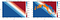Figure 7 A NN with only one neuron after the input layer produces a linear decision boundary. The shading of the background in the 2D feature space shows the probability for a fake banknote. On the right side, the training data are overlaid, showing that the linear decision curve doesn’t fit nicely in the boundary between the real and fake banknotes.

The shading of the background in figure 7 indicates the predicted probability for an instance with the corresponding values of the two features. The white color indicates positions in the feature space where the probability for both classes is 0.5. Points on the one side are classified to one class and points on the other side, to the other class. This boundary is called the decision boundary. As you can see, it’s a line. This isn’t a coincidence but a general property of a single artificial neuron with a sigmoid as activation function, also known as logistic regression. In a 2D features space, the decision boundary is a straight line. It isn’t curved and has no wiggles. If you have three features, the boundary is a plane (no wiggles), and it stays an object with no wiggles for a feature space with more than three dimensions, which is called hyperplane.

In the banknote example, the true boundary between the two classes is curved. Therefore, a single neuron isn’t appropriate to model the probability for a fake banknote based on its two features. To get a more flexible model, we introduce an additional layer between the input and output layers (see figure 8). This layer is called the hidden layer because its values aren’t directly observed but are constructed from the values in the input layer.

In this example, the hidden layer holds eight neurons; each gets as input a weighted sum of the same input features but with different weights. The weighted sum is then transformed by the activation function. You can think about these neurons in the hidden layer as a new representation of the input. Originally, it was represented by two values (features), now it’s represented by eight values (features) — the output of the eight neurons. This is sometimes called feature expansion. You can use different numbers of neurons in the hidden layer, which is part of the design of the NN.

The output layer gives the probability for the instance to be a real or fake banknote. You have seen that one neuron is sufficient in a binary classification problem because knowing the probability p of one class fixes the probability of the other class to 1 — p. You can also use two neurons in the output layer: one neuron modeling the probability for the first class and the other neuron modeling the probability for the second class. This output layer design generalizes to classification tasks with more than two classes. In that case, the output layer has as many neurons as you have classes in the classification problem. Each neuron stands for a class, and you want to interpret the output of the neuron as the probability for the class. This can be done using the softmax function. The softmax function takes the weighted sum zi and transforms it into a probability pi by setting

This ensures that the values are between zero and one and further add up to one. You can therefore interpret pi as the probability for the class i. The soft in softmax indicates that, rather than giving a hard call to one of the possible classes, the network can assign smaller probabilities to the other classes.

The y vector of the training data also has to be changed to be compatible with the two outputs. It was y = 1 if the example belonged to the class fake and y = 0 for the class real. Now you want the label to describe the two possible outputs. A real banknote should have the output values p0=1 and p1=0, and a fake banknote, the values p0=0 and p1=1. This can be achieved by a one-hot encoding of y. You start with a vector with as many zeros as you have classes (here two). Then you set one entry to 1. For y = 0, you set the 0th entry to 1, for y = 1 the first. For the architecture of the fcNN, see figure 2.8 and for the corresponding Keras code, see Listing 2.2.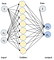Figure 8 An fcNN with one hidden layer consisting of eight nodes. The input layer has two nodes corresponding to two features in the banknote data set, and the output layer has two nodes corresponding to two classes (real and fake banknote).

Listing 2 Definition of the network with two hidden layers

`model = Sequential() model.add(Dense(8, batch_input_shape=(None, 2),                           activation=’sigmoid’)) ❶  model.add(Dense(2, activation='softmax'))        ❷  # compile model model.compile(loss='categorical_crossentropy',                       optimizer=sgd)`

❶ Definition of the hidden layer with eight neurons

❷ The output layer with two output neurons

As you can see in figure 9, the network now yields a curved decision surface, and it’s better able to separate the two classes in the training data.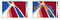Figure 9 An fcNN produces a curved decision boundary. The shading of the background in the 2D feature space shows the probability for a fake banknote which was predicted by an fcNN with one hidden layer containing eight neurons using the features x1 and x2 as input. On the right side, the training data are overlaid, showing that the curved decision boundary much better fits the boundary between the real and fake banknotes.

Add more hidden layers in the banknote notebook https://github.com/tensorchiefs/dl_book/blob/master/chapter_02/nb_ch02_01.ipynb and you become a member of the DL club. It’s much easier than machine learning (see figure 10).

But what’s going on when adding an additional layer? In principle, the same thing as we’ve discussed for the first hidden layer. You can see the neuron values in the added hidden layer as a new feature representation of the input, but there’s one difference: the features in deeper layers aren’t directly constructed from the input but from the previous layer. For example, in the second hidden layer, the features are constructed from the features in the first hidden layer (see figure 12). This hierarchical construction of the features is often efficient because it allows you to learn from the first layer basic features that can be used as components in several more complex features of the next layer.

By stacking many layers together, you allow the NN to construct hierarchical and complex features that get more and more abstract and task specific when going from layer to layer. As the number of neurons per layer (and also the number of hidden layers) is part of the design, you need to decide if this number, for example, is based on the complexity of your problem and your experience, or if it’s what’s reported by other successful deep learners.

The good news in DL is that you don’t need to predefine weights that determine how to construct the features in one layer from the features in the previous layer. The NN learns this during the training. You also don’t need to train each layer separately, but you usually train the NN as a whole, which is called end-to-end training. This has the advantage that changes in one layer automatically trigger adaptations in all other layers.

That’s all for now. If you want to learn more about the book, check it out on liveBook here.